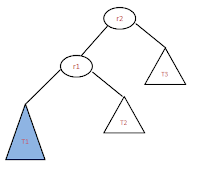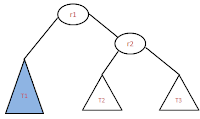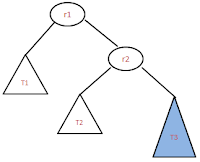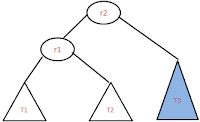## Friday, August 24, 2012

### AVL tree Single rotation!!

Single rotation: As the name says need to do one rotation (left or right) to balance the tree. In this there are two types of sub rotations
• Left left rotation
• Right right rotation
﻿﻿Left Left rotation: This is single rotation method which needs only one rotation. Left-Left rotation is required if the un-balanced tree is like as shown in the image.
﻿﻿Before Rotation
• Here r2  is un-balanced, because of adding the new node at T1 sub tree. Newly added node could either left or right. But it changes the balance property of r2.
• To balance the tree, we need to do left-left rotation. This is because of newly added node is left child of r1 and r1 is a left child of un-balanced node r2.
• Rotation is done in such a way that T1<r1<T2<r2<T3 property should satisfy after the rotation also.
•After Rotation
After the rotation the tree would be as shown in the image.
• To make the tree balance, make the r2 as the root, and follow the Binary search tree property after that, we will get the resulted tree.
• Sample code to do left-left rotation
```r1 = r2->left;
//we are @r2, below statements are to make the tree like
// shown in the image. so that rotation becomes easy
t1 = r1->left;
t2 = r1->right;
t3 = r2->right;

//actual rotation happens here
r1->right = r2;
r2->left = t2;

// update the r1 , r2 height
set_height(r1);
set_height(r2);
```

Right Right rotation: This is single rotation method which needs only one rotation. Right-Right rotation is required if the un-balanced tree is like as shown in the image.

﻿Before Rotation
﻿
• Here r1 is un-balanced, because of adding new node to t﻿﻿he subtree T3 either left or right. But it changes the balanced property of r1.
• We need to do right-right rotation because newly addded node is right side of r2, and r2 is right side of the unbalanced node r1.
• Rotation is done in such a way that T1<r1<T2<r2<T3 property should satisfy after the rotation also.
•After Rotation
• After the rotation, resulted tree shouls be as shown in the image.
• To make the tree balance, make the r2 as the root, and follow the Binary search tree property after that, we will get the resulted tree.
• See the sample code for right-right rotation below.
```
//we are @r1 and below statements are
// used to arrange the pointers looks like in the image
r2 = r1-<right;
t1 = r1-<left;
t2 = r2-<left;
t3 = r2-<right;

// actual rotations happens here
r1-<right = t2;
r2-<left = r1;

set_height(r1);
set_height(r2);

*r = r2;

```